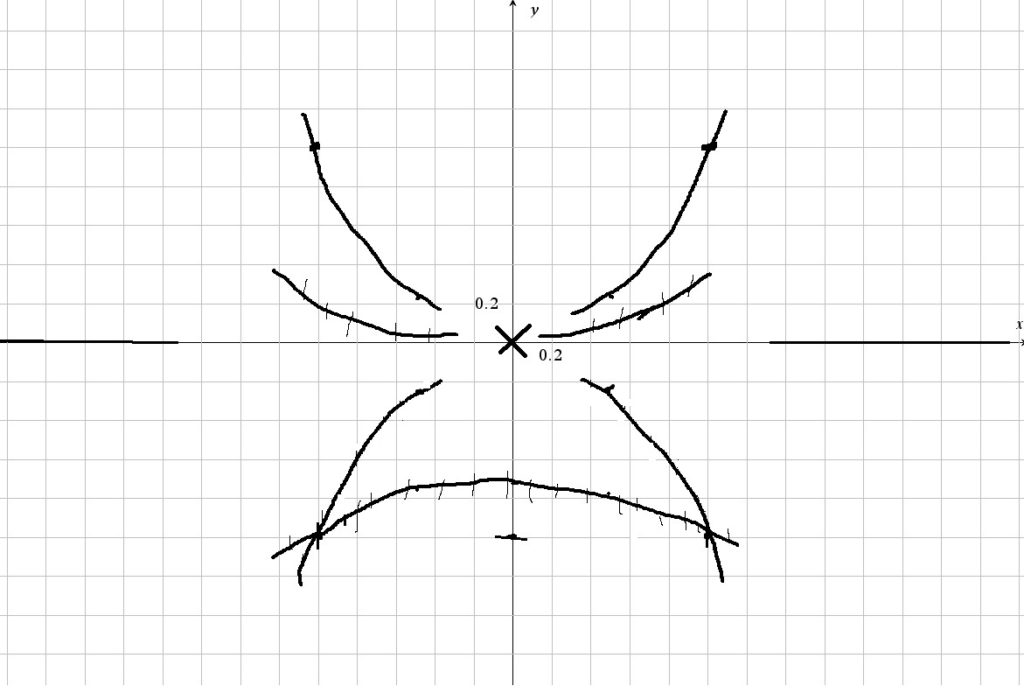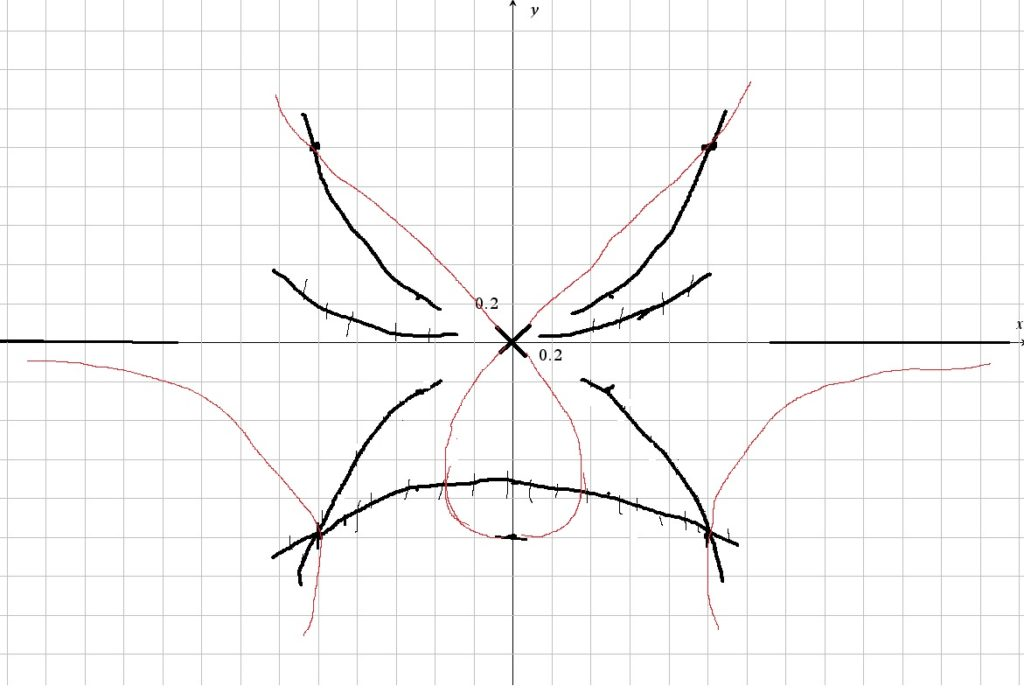# y^3+y^2=(x^4)y+x^2

Up a level : Differentiation, derivatives
Previous page : y^3+y^3=x^2+x
Next page : y^3+x^2=y/xLet us look at${y^3} + {y^2} = {x^4}y + {x^2}$

We can immediately see that the point (0, 0) is on the graph.

Zeroes

If y=0 we get$0 = {x^2}$

So we have, the already known, zero at x=0

If x=0 we get${y^3} + {y^2} = 0$

So we have y-intercepts at y=0 and y=−1.

So we have the points (0, 0), again, and (0, −1).

Asymptotes

Say y goes toward infinity then we basically have${y^3} = {x^4}y$

or${y^2} = {x^4}$

and thus$y = \pm \sqrt {{x^4}} = \pm {x^2}$

So we should expect that y=x2 and y=−x2 to be asymptotes.

What if x goes toward infinity? This is quite less obvious, at least to start with, since we have the term x4y.  But if x and y are very small we get that${y^3} + {y^2} = {x^4}y + {x^2}$

will behave as${y^2} = {x^2}$

or$y = \pm x$

We could thus expect the graph to cross itself at the origin, and that the two parts will have slopes of 1 and −1 respectively.

Intersection between then asymptotes and the relation

Since we have an x2 term in the relation it might be an idea to see if the substitution$y = \pm {x^2} \Rightarrow {x^2} = \pm y \Rightarrow {x^4} = {y^2}$

We get${y^3} + {y^2} = {y^2}y \pm y = {y^3} \pm y$

or$y = \pm 1$

So we now know we have points at (±1, ±1)

Horizontal tangent points, i.e. stationary points

We may find these by finding where y´=0. So we take the implicit derivative of our relation to get$3{y^2}y' + 2yy' = 4{x^3}y + {x^4}y' + 2x$

Here we needed both the chain rule and the product rule. For y´=0 this gives us$0 = 4{x^3}y + 2x$

This is a bit problematic, since we have both x and y in the equation. We could make y the subject and substitute that into the original equation, but that would give us an eight degree polynomial to solve, so let us instead make y´ the subject to see what slopes we have at the known points. We get$3{y^2}y' + 2yy' - {x^4}y' = 4{x^3}y + 2x$

Or$y'(3{y^2} + 2y - {x^4}) = 4{x^3}y + 2x$

And thus$y' = \frac{{4{x^3}y + 2x}}{{3{y^2} + 2y - {x^4}}}$

For the point (0, −1) it gives us$y' = \frac{0}{1} = 0$

For the point (0, 0) gives us that the derivative is undefined – as is expected.

For the vertical tangent points we get into the same problem as with the horizontal slopes. We get an expression for the slope that is dependent on both x and y.  We get that$3{y^2} + 2y - {x^4} = 0$

Could it be that any of our known points satisfies this? We know (0,0) is a point where the graph intersects itself, and that the graph is horizontal at (0,-1).  But how about the (pm1,pm1). The sign of the x-coordinate cannot matter, since we take that to the power of four. So, let us test$3{y^2} + 2y - {x^4} = 0$

For this to be true y=−1. So we have that the graph is vertical at (1, −1) and (−1,−1).

We could also have made y the subject of$3{y^2} + 2y - {x^4} = 0$

To get$y = \frac{{ - 2 \pm \sqrt {{2^2} + 4 \cdot 3{x^4}} }}{{2 \cdot 3}} = \frac{{ - 1 \pm \sqrt {1 + 3{x^4}} }}{3}$

So any possible points where the graph is vertical could be along the two lines given by the above equation. We could calculate this for a few points and connect the points. For x=0 we get y=0 and y=-2/3, for x=1 we get y=1/3 and y=-1 (as expected). For x=1/2 we get$y = \frac{{ - 1 \pm \sqrt {1 + \frac{3}{{16}}} }}{3} \approx \frac{{ - 1 \pm (1 + \frac{3}{{16}})}}{3} = - \frac{1}{3} \pm \frac{1}{3} \pm \frac{1}{{16}}$

I.e. just slightly above 0 or just slightly below −2/3.

Putting it all together (using paint, and the mouse (implies ugly)). I getThe dark lines are either asymptotes, or, if they have vertical lines on then, they are places where one could possibly have vertical stretches of the graph. So, next I tried to draw some kind of graph that could fulfil this (it would look rather much better on a piece of paper).So, how does it compare to the actual graph?Up a level : Differentiation, derivatives
Next page : y^3+x^2=y/xLast modified: Mar 20, 2022 @ 13:19